# Text Overlay on an Image, And Save using MATLAB : Add text over an Image & Save Using MATLAB

To the people who love MATLAB, especially for accomplishing the task of image processing it may happen that editing or enhancing the image is felt more comfortable in MATLAB as compared to  photo editing software like Picasa, Paint, or even Photoshop!

This time we just want to overlay some text using MATLAB over an image. In MATLAB its a 4 line command work (or you may even further reduce it).

So the MATLAB code for Text Overlay on an Image, And Save is:

text(20, 300, 'Divilabs.com', 'FontName', 'Comic Sans MS', 'FontSize', 20, . . .
'BackgroundColor', [.6, .6, .6], 'VerticalAlignment', 'Bottom'); %all the text option to try out with

a = getframe(gcf); %getting a screen shot for the currently opened plot window

imwrite(a.cdata, 'resultant.jpg'); %saving the text overlayed image file

Explanation of the above MATLAB code:
Here in the above code we are reading an image & loading it on an image plot window. Then the 'text' function will write the text over the plotted image in that window itself.
The first 2 parameters 20 & 300 are just to specify the location of overlayed text on the image. the next 4 parameters 'FontName', 'Comic Sans MS', 'FontSize', 20 are obviously suggesting the font name & size to the text function.
What the parameter set 'BackgroundColor', [.6, .6, .6] will do is, they will specify the background color on which the text will be written in the image. 'VerticalAlignment', 'Bottom' will write the text on the bottom of that text box.

a = getframe(gcf) : this will click a screen shot of the current image plot window with the text overlayed.

imwrite(a.cdata, 'resultant.jpg'): this will save the image file with the text overlayed with the name resultant.jpg

Resultant Images with text overlayed on tweaking with different Argument of text function

With the command set:
text(20, 300, 'Divilabs.com', 'FontName', 'Comic Sans MS', 'FontSize', 20, ...
'BackgroundColor', [.6, .6, .6], 'VerticalAlignment', 'Bottom');

We got:Text Overlay on an Image, And Save using MATLAB : Add text over an Image & Save Using MATLAB
With the command set:
text(50, 400, 'Divilabs.com', 'FontName', 'Comic Sans MS', 'FontSize', 25, ...
'BackgroundColor', [.8, .6, .8], 'VerticalAlignment', 'Center');

We got:Text Overlay on an Image, And Save using MATLAB : Add text over an Image & Save Using MATLAB
So, at last I would suggest that just play around by tweaking the above parameters & get the desired result. If you got some interesting result please do add that in the follow up comments below or just mail be so that I could add up in the article.

# Contrast Stretching or Contrast Normalization an Image in MATLAB

Contrast stretching is a simple image enhancement technique that improves the contrast in an image by expanding the dynamic range of intensity values it contains. It can only apply a linear scaling function to the image pixel values.

## Some Mathematical Modelling: (source: ed.ac.uk)

It is necessary to specify the upper and lower pixel value limits over which the image is to be normalized. Often these limits will just be the minimum and maximum pixel values that the image type concerned allows. For example for 8-bit graylevel images the lower & upper limits might be 0 and 255. Call the lower and the upper limits a and b respectively.
The simplest of stretching scans the image to find the lowest & highest pixel values in the image. Call these c and d. Then each pixel P is scaled using the following function: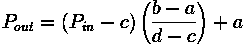Values below 0 are set to 0 and values about 255 are set to 255.

MATLAB implementation of the above concept of Contrast Stretching or Contrast Normalization on an image is:
figure(1);
imshow(X);
title('Original Image')
a = min(X(:));
%minimum pixel of image X
b = max(X(:));
%maximum pixel of image X
X= (X-a).*(255/(b-a));
%just using the formula above
figure(2);
imshow(X);
title('Contrast Streached Image')

Results of Contrast Stretching in MATLAB:The original Image: Not Contrast StretchedThe Resultant Image: Contrast Stretched

# Removing the leading & trailing zeros from a vector Using MATLAB

This is what we need while we are developing in communication systems. These zeros could be used just to gather synchronization information or they may act as a start or end delimiter of the transmitted frame.
Thus to get the useful data back we need to remove all these padded zeros from the received frame, which could be visualized by a vector in MATLAB.

Removing both leading & trailing zeros from a vector Using MATLAB
Suppose we are having a vector like:
x=[0 0 0 -4 -2 0 -7 -8 0 0 0]
Clearly it has been padded by three zeros at the end & the starting.
But How to remove that? And that too specifically by using our Favorite software MATLAB!

So to get the vector as 4 -2 0 -7 -8 what we are going to do is type the below statement,

y = x(find(x,1,'first'):find(x,1,'last'));

The above will remove all the leading & trailing zeros from the vector 'x' & store it in vector 'y'.
Result,
y =

-4    -2     0    -7    -8

Removing only leading zeros from a vector Using MATLAB
What if we just have padded only the leading zeros & trailing zeros are actually important bits of information, so we just need to remove the leading zeros from the vector 'x'.
How we are going to do it is simply like typing the following command,

y=x(find(x, 1, 'first'):end)

& will result in,
y =

-4    -2     0    -7    -8     0     0     0

Removing only trailing zeros from a vector Using MATLAB
At last what if we want only the trailing zeros to be removed from the vector. Another simple single line MATLAB code for that will be,

y=x(1:find(x, 1, 'last'))

& will result in
y =

0     0     0    -4    -2     0    -7    -8

So, it was a simple stuff. More specifically it was a game of array indexing of/in MATLAB.
You just going some experience in MATLAB to get an idea of these small but beautiful tricks!

# MATLAB Program for Converting a Text File to Stream of Bits

What is a text file specifically? A sequence of character.
And how these characters can be visualized in terms of a number? By their ASCII equivalent.
And the ASCII equivalent code can be converted to binary format to get bit stream.
Refer WikiPedia page of ASCII to get a detailed information about ASCII codes. Or search over the internet with the term "ASCII".

The following code will be a blend of MATLAB's data file handling (accessing & reading the file saved in disk), some processing & interconversion among data-types (typecasting to be more specific).

The resultant 1D array will be a continuous stream of  1s & 0s depending on the text in the text file.

MATLAB code for Converting a Text File to Stream of Bits

clc
clear all
close all
[f_name,path] = uigetfile({'*.txt';'*.*'},'Select the Text (.txt) file');

%above line will let you to browse for the file using a GUI window
path=[path f_name]; %building the path of the text file
A = importdata(path);
%import a text data as a structure
x=A(1);
for i=2:size(A,1)
x=strcat(x,'\n');
%preserving a line brake like this, it will be helpful while reconstruction
x=strcat(x, A(i));
end
%this loop is to concatenate all the characters in a continuous sequence
x=x{1}; %converting structure to a character array
c=dec2bin(x,8); %create a cell of x character & 8bit
y=c(1,:);
%for a continoues bit stream
for i=2:size(c,1)
y=strcat(y,c(i,:));
end
for i=1:size(y,2)
z(i)=double(y(i));
end
z(find(z(:)==48))=0;
z(find(z(:)==49))=1;
%z is a bitstream now
disp('The Resultant Bit Stream is:');

disp(z);

Sample Text & the output bit stream using above MATLAB code to convert a text file to bit stream.

Suppose the text file is with the name : test.txt contains the following text,
Orissa
rajashthan
Uttrakhand
bihar
Gujrat
Andaman and nicobar

The resultant bit stream after the execution is:
010000010110111001100100011010000111001001100001001000000111000001110010011
000010110010001100101011100110110100001011100011011100100111101110010011010
010111001101110011011000010101110001101110011100100110000101101010011000010
111001101101000011101000110100001100001011011100101110001101110010101010111
010001110100011100100110000101101011011010000110000101101110011001000101110
001101110011000100110100101101000011000010111001001011100011011100100000101
110010011101010110111001100001011000110110100001100001011011000010000001110
000011100100110000101100100011001010111001101101000010111000110111001010100
011000010110110101101001011011000010000001001110011000010110010001110101010
1110001101110010001110111010101101010011100100110000101110100010111000110111
0010000010110111001100100011000010110110101100001011011100010000001100001011
0111001100100001000000110111001101001011000110110111101100010011000010111001
0010111000110111001001101011000010110010001101000011110010110000100100000010
10000011100100110000101100100011001010111001101101000

# QPSK Digital Modulation Simulation Using MATLAB: Quadrature Phase Shift Keying

Quadrature Phase Shift Keying (QPSK) is one of  the type of phase shift keying. Unlike BPSK which send one bit information at an instance, QPSK is a modulation which sends two bits of digital information a time (without the use of another carrier frequency). They both are by the way DSBCS modulation scheme.

In a sense, QPSK is an expanded version from binary PSK where in a symbol consists of two bits and two orthonormal basis functions are used. A group of two bits is often called a 'dibit'. So, four dibits are possible. Each symbol carries same energy.

The above was just in introduction about what QPSK is, search over the internet to get more stuffs about it. You will get number of learning resources which explains you the A-Z of the theoretical aspect of it.

The process which we have followed while implementing on MATLAB is quite conventional.
First: get the bit stream.
Then Divide the bit stream in 2 bit streams: even & odd.
Then apply the BPSK techniques on these 2 bit streams separately.
Add the modulated waves to get the QPSK.

Another way to implement it in MATLAB or any other simulation software would be, to introduce a phase shift of  90 degree, on each pair of bit change. For more information just search Maxim Integrated QPSK tutorial, here you will get all the relevant equation information.

MATLAB Code For QPSK :

clc;
clear all;
close all;
cvx=input('Enter Length of Random Bit Sequence:');
d=round(rand(1,cvx))
l=cvx;
x=0:0.01:l*2*pi;
cc=cos(x);
cs=cos(x+pi/2);
k=length(cc);
k1=k/l;

for i=1:l
if(d(i)==0)
d(i)=-1;
i=i+1;
end
end

i=1;
j=1;
% To segrigate odd bit streams and even bit streams
while (i<l) && (j<l)
%half strem with double symbol duration
dd1(j)=d(i);
dd1(j+1)=d(i);
dd2(j)=d(i+1);
dd2(j+1)=d(i+1);
j=j+2;
i=i+2;
end
% to make bit streams cycle equivalent to sinusoidal waveformt=1;
for i=1:l
for j=1:k1
%k1 sample with 1 sine period
dd(t)=d(i);
d1(t)=dd1(i);
d2(t)=dd2(i);
t=t+1;
j=j+1;
end
i=i+1;
end

subplot(6,1,1);
stairs(dd);
axis([0 t -2 2]);
title('Imput Bit Stream');
subplot(6,1,2);
stairs(d1);
axis([0 t -2 2]);
title('Odd Bit Stream');
subplot(6,1,3);
stairs(d2);
axis([0 t -2 2]);
title('Even Bit Stream');

len=length(d1);
if(k<len)
len=k;
end

for i=1:len
qcc(i)=cc(i)*d1(i);
% odd streams multiplied with I waveform
qcs(i)=cs(i)*d2(i);
% even streams multiplied with Q waveform
i=i+1;
end

subplot(6,1,4);
plot(qcc);
axis([0 len -2 2]);
title('Modulated Wave of I-Component');
subplot(6,1,5);
plot(qcs);
axis([0 len -2 2]);
title('Modulated Wave of Q-Component');

qp=qcc+qcs;
subplot(6,1,6);
plot(qp);
axis([0 len -2 2]);
title('QPSK WAVEFORM');
figure, scatter(dd1,dd2,40,'*r');
title('Constellation Diagram of QPSK');

Results: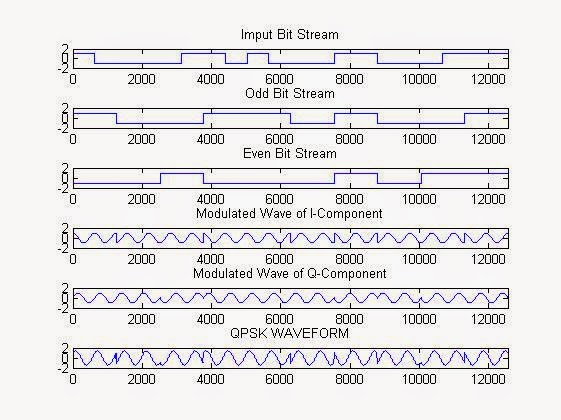QPSK MATLAB Simulation Result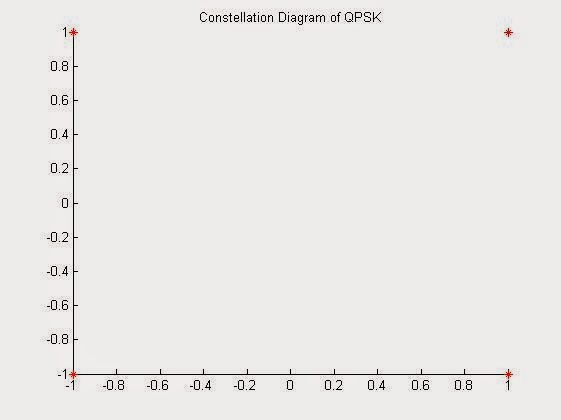QPSK Constellation Plot

# Sound Record, Save & Playback in MATLAB

Yes Matlab is also capable to handle the devices that are connected to the computer system ports. It can gather data from these devices.
In this example we will gather sound data from the microphone connected to your system. The code is optimized for the system which is having more than one audio input device connected to them. In that case, although from a single instance of the code run you will be able to collect data only from one of the device (will be selected though the device id). But if run multiple instance of MATLAB with the same code you will be able to record simultaneously from different input devices.

The following program will require you to select the device id, set the duration of the recoding, & the bit depth of recording during runtime.
The recording is happening in the sampling rate of 48kHz & dual channel, i.e., stereo. The audio data will be stored by the name 'xyz.wav'.

NOTE: you can select different sampling rate, that is supported by your recording device (or sound card) just see the microphone properties in "Control Panel".

MATLAB code for  Sound Record, Save & Playback:

clc;
clear all;
close all;
t=audiodevinfo;
%getting audio device status connected with the computer system
n=size(t.input,2); %getting especially the input device status
disp('Details Of Audio Recording device Connected:');
for i=0:n-1
disp('Device Name:')

disp(t.input(1,i+1).Name) %display the audio input device name
disp('Device id:')
disp(i)
%diaplay the device id
end
d=input('Enter your Device ID from the above Device List to Start Recording:');
%getting the device id for recording
t=input('Enter the Duration of Recording(s):');
%getting the duration of recording
b=input('Enter Bit Depth of Recording(e.g. 8bit):'); %getting the bit depth of recording
disp('Recording at 48kHz Sampling frequency');
aud=audiorecorder(48000,b,2,d);
disp('Recording....')
recordblocking(aud,t);
myRecording = getaudiodata(aud);
%start audio recoding
plot(myRecording); %plot the recorded data
audiowrite('xyz.wav',myRecording, 48000); %write the recorded audio to a file
disp('Saving the Audio File as xyz.wav in the working directory...!');
disp('Playing....');
play(aud);
%play the recorded file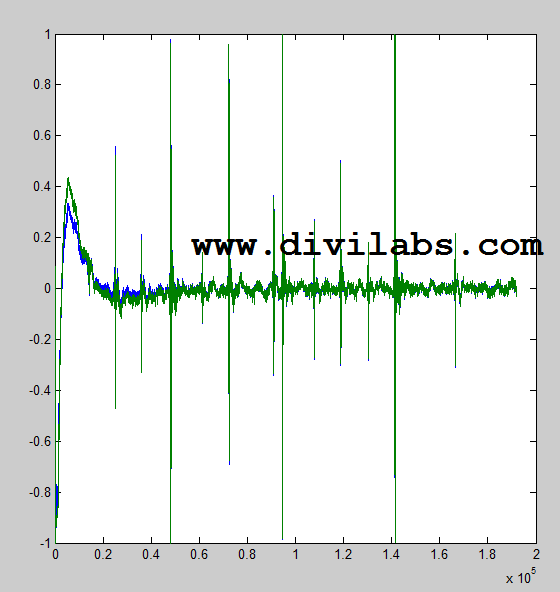Sound Record Plot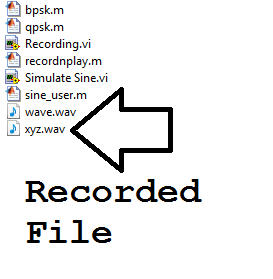Recorded File: As seen on Explorer for the current working directory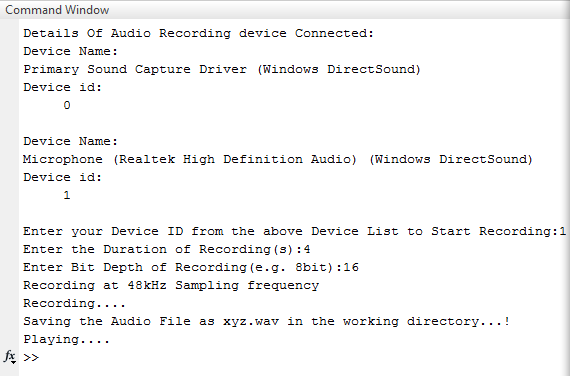Audio Recorder in Action